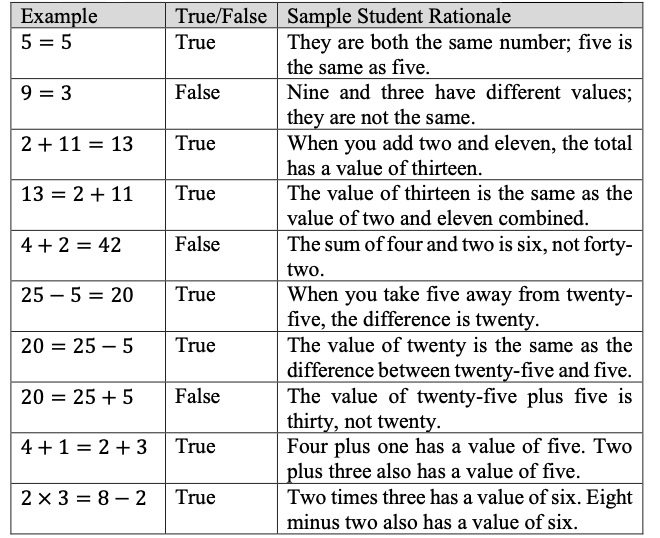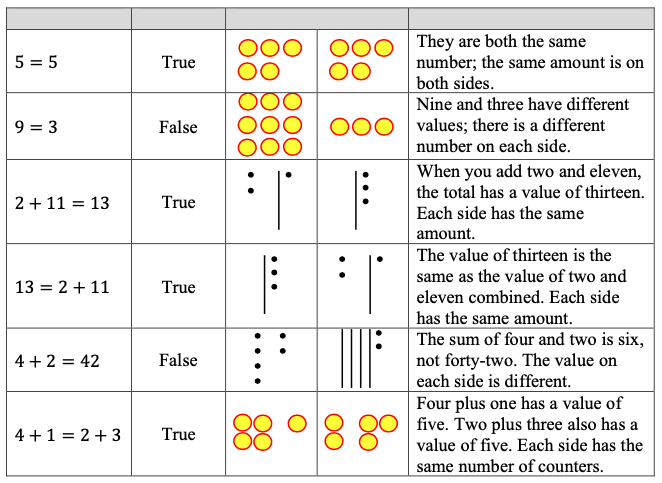# Standard #: MA.3.AR.2.2

This document was generated on CPALMS - www.cpalms.org

Determine and explain whether an equation involving multiplication or division is true or false.

### Examples

Given the equation 27÷3=3×3 , it can be determined to be a true equation by dividing the numbers on the left side of the equal sign and multiplying the numbers on the right of the equal sign to see that both sides are equivalent to 9.

### Clarifications

Clarification 1: Instruction extends the understanding of the meaning of the equal sign to multiplication and division.

Clarification 2: Problem types are limited to an equation with three or four terms. The product or quotient can be on either side of the equal sign.

Clarification 3: Multiplication is limited to factors within 12 and related division facts.

### General Information

Subject Area: Mathematics (B.E.S.T.)
Strand: Algebraic Reasoning
Status: State Board Approved

• Equation
• Expression
• Equal sign

### Vertical Alignment

Previous Benchmarks

Next Benchmarks

### Purpose and Instructional Strategies

The purpose of this benchmark is to extend the understanding of the meaning of the equal sign in multiplication and division situations. In Grades 1 and 2, students determined and explained when addition and subtraction equations were true or false (MA.1.AR.2.2, MA.2.AR.2.1).
• Instruction should emphasize that the equal sign can be read as “ the same as” to show the balance of two multiplication and/or division expressions. When those expressions are evaluated as the same product or quotient, the equation is balanced, or true. If those expressions evaluate differently, then the equation is not balanced, or false (MTR.2.1, MTR.5.1).
• When students explain whether an equation is true or false, they should justify by explaining the equivalence of its expressions. (Note: The expectation of this benchmark is not to compare the expressions of a false equation using symbols of inequality, < or >.) (MTR.4.1, MTR.6.1)

### Common Misconceptions or Errors

• By Grade 3, students may grow to expect equation solutions to be represented as the expressions on the right side of the equal sign. When having students evaluate true or false equations with only three terms (e.g., 18 = 3 × 6), teachers should give examples showing products and quotients on the left side.

### Strategies to Support Tiered Instruction

• Instruction includes opportunities to explore the meaning of the equal sign. The teacher provides clarification that the equal sign means “the same as” rather than “the answer is.” Multiple examples are provided to evaluate equations as true or false using the four operations with the answers on both the left and right side of the equation, beginning by using single numbers on either side of the equal sign to build understanding. The same equations are written in different ways to reinforce the concept.
• For example, the teacher shows the following equations, asking students if they are true or false statements. Students explain why each equation is true or false, repeating with additional true and false equations using the four operations.• Teacher provides opportunities to explore the meaning of the equal sign using visual representations (e.g., counters, drawings, base-ten blocks) on a t-chart to represent equations. The teacher provides clarification that the equal sign means “the same as” rather than “the answer is.” Multiple examples are provided for students to evaluate equations as true or false using the four operations with the answers on both the left and right sides of the equation, beginning by using single numbers on either side of the equal sign to build understanding. The same equations are written in different ways to reinforce the concept.
• For example, the teacher shows the following equations. Students use counters, drawings, or base-ten blocks on a t-chart to represent the equation. The teacher asks students if they are true or false statements and has them explain why each equation is true or false, repeating with additional true and false equations using the four operations.Two equations are below. One equation is true, and the other equation is false. Choose one of the equations and explain why it is true or false.
2 × 3 = 4 × 6
2 × 12 = 4 × 6

### Instructional Items

Instructional Item 1

Which of the following describes the equation 16 ÷ 2 = 36 ÷ 9 ?
• a) This equation is true because the expressions on each side have a quotient of 8.
• b) The equation is true because the expressions on each side have a quotient of 4.
• c) This equation is false because the expressions on each side have a quotient of 8.
• d) This equation is false because the quotient on the left is 8 and the quotient on the right is 4.

*The strategies, tasks and items included in the B1G-M are examples and should not be considered comprehensive.

#### Related Courses

 Course Number1111 Course Title222 5012050: Grade Three Mathematics (Specifically in versions: 2014 - 2015, 2015 - 2022, 2022 and beyond (current)) 7712040: Access Mathematics Grade 3 (Specifically in versions: 2014 - 2015, 2015 - 2018, 2018 - 2022, 2022 and beyond (current)) 5012055: Grade 3 Accelerated Mathematics (Specifically in versions: 2019 - 2022, 2022 and beyond (current)) 5012015: Foundational Skills in Mathematics 3-5 (Specifically in versions: 2019 - 2022, 2022 and beyond (current))

#### Related Access Points

 Access Point Number Access Point Title MA.3.AR.2.AP.2 Determine if multiplication or division equations with no more than three terms are true or false. Multiplication may not exceed two single-digit whole numbers and their related division facts.

#### Formative Assessment

 Name Description Does It Work for Division? Students are asked if the Commutative Property holds for division.

#### Lesson Plan

 Name Description Arrays Show the Way to the Multiplication Chart This is an introductory lesson to explore the use of arrays to solve multiplication problems. Students build arrays and save the arrays in a class Multiplication Chart. They learn to use arrays to find products and factors, and by placing them in the Multiplication Chart, they learn how to read the chart. They learn how to write equations to represent situations that are modeled with arrays. An overall theme is the organization of the multiplication chart and how it includes arrays within.

#### Perspectives Video: Teaching Idea

 Name Description Equations on the Math Balance Unlock an effective teaching strategy for teaching inequalities and equations with the math balance in this Teacher Perspectives video for educators.
Printed On:12/4/2023 5:42:19 AM
Print Page | Close this window